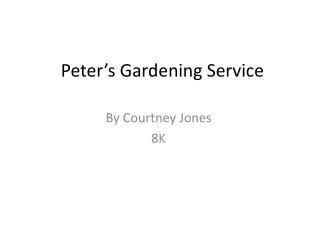Download PresentationPeter’s Gardening Service

Loading in 2 Seconds...

# Peter’s Gardening Service - PowerPoint PPT Presentation

Peter’s Gardening Service. By Courtney Jones 8K. Investigation. Peter is a professional gardener who tends the gardens and lawns of his many clients. One of his jobs is to fertilise the lawns regularly. He is trying a new fertiliser which, according to the directions,I am the owner, or an agent authorized to act on behalf of the owner, of the copyrighted work described.
Download Presentation## Peter’s Gardening Service

An Image/Link below is provided (as is) to download presentation

Download Policy: Content on the Website is provided to you AS IS for your information and personal use and may not be sold / licensed / shared on other websites without getting consent from its author.While downloading, if for some reason you are not able to download a presentation, the publisher may have deleted the file from their server.

- - - - - - - - - - - - - - - - - - - - - - - - - - E N D - - - - - - - - - - - - - - - - - - - - - - - - - -
Presentation Transcript
1. Peter’s Gardening Service By Courtney Jones 8K

2. Investigation Peter is a professional gardener who tends the gardens and lawns of his many clients. One of his jobs is to fertilise the lawns regularly. He is trying a new fertiliser which, according to the directions, states that when the contents of the container are mixed with water the solution covers 64m2 of lawn. To help him decide how many containers of fertiliser he may require, Peter needs to have some idea of possible sizes of lawn one container will cover. First Peter considers a square lawn. He wants to find the length of a square with area 64m2.

3. Questions 1) If the length of the square is represented by x, write an expression for the area of the square. 2) Since the area needs to be 64m2, write an equation which shows that the area of the square is equal to 64m2 using x. Part 1 will help. 3) Can you solve this equation? Remember to consider the different methods you have learnt. (You may wish to discuss this with a partner or with others in a small group.) What is the size of a square lawn which can be covered with one container of fertiliser?

4. Square Lawn Question one: Area = x² x Question two: x= √ of 64 x Question three: X = 8m

5. Rectangular Lawn 1. If the length of the rectangle is represented by l, and the width is represented by w, write an expression for the area of the rectangle 2. Since the area needs to be 64m², write an equation which shows the area of the rectangle is equal to 64m² using l and w. 3. Can you solve this equation? What are 5 different sizes of a rectangle lawn which can be covered by one tin of fertiliser? • L x W = Area • 64m² = L = W • a. 64m ÷ 1m = 64m² Lb. 64m ÷ 2m = 32m²c. 64m ÷ 4m = 16m²d. 64m ÷ 5m = 12.8m² we. 64m ÷ 10m = 6.4²

6. Triangle Lawn • 1. If the height of the triangle is represented by h, and the base is represented by b, write an expression for the area of the triangle. • 2. Since the area needs to be 64m², write an equation which shows the area of the triangle is equal to 64m² using h and b. • 3. Can you solve this equation? What are 5 different sizes of a triangle lawn which can be covered by one tin of fertiliser? • 1. 0.5 x h x b = Area • 2. 64m² ÷ (0.5 x h) = b • 3. a. 64m ÷ (0.5 x 2m²) = 64m² • b. 64m ÷ (0.5 x 10m²) = 12.6m² • c. 64m ÷ (0.5 x 20m²) = 6.4m² • d. 64m ÷ (0.5 x 8m²) = 8m² • e. 64m ÷ (0.5 x 32m²) = 4m² b h

7. Circular Lawn 1. If the radius of the circle is represented by r, write an expression for the area of the circle. 2. Since the area needs to be 64m², write an equation which shows that the area of the circle is equal to 64m² using r. 3. Can you solve this equation? What is the size of a circle lawn which can be covered by one tin of fertiliser? 1. Π x r² = Area 2. 64m²  Π= r² - √(64m²  Π) = r 3. 64m²  Π= r² - √(64m²  Π) = r - 4.51m² = r r

8. L Shaped Lawn 1. If the length of the l-shape is represented by l, write an expression for the area of the l-shape. 2. Since the area needs to be 64m², write an equation which shows that the area of the l-shape is equal to 64m² using l. 3. Can you solve this equation? What is the size of a l-shaped lawn which can be covered by one tin of fertiliser? 1. l² x 3= Area L 2. √(64m² 3) = l 3. √21.33m² = l - 4.62m² = l L L L